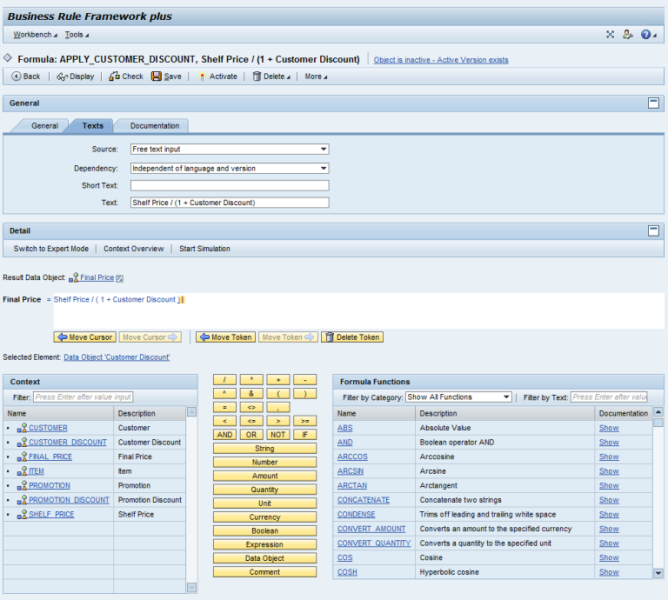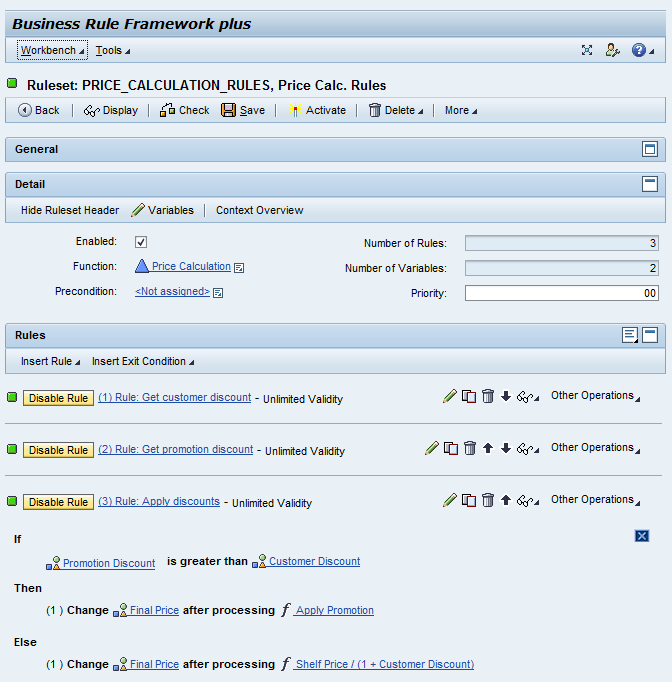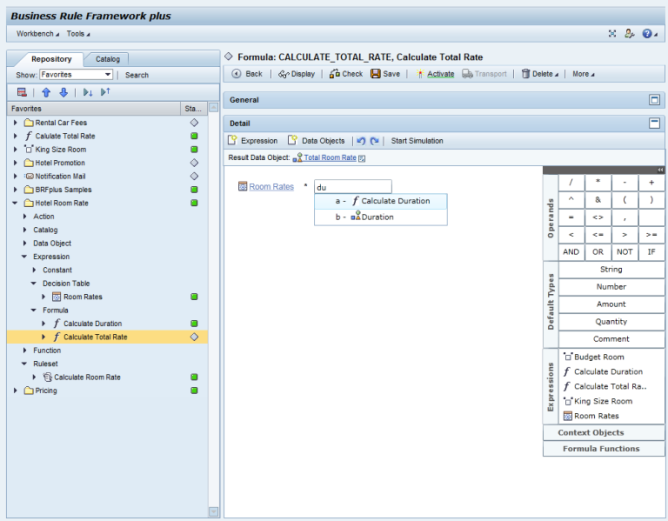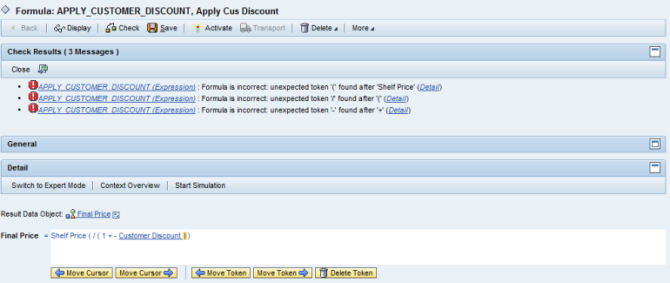# News in the BRFplus Formula

Customer feedback shows that BRFplus formula expressions are heavily used. Next to decision tables they are probably the most popular concept.
Often customers do not make use of texts to simplify the user experience. In case of formulas, texts could be used to show the particular calculation step. For example take a look at the following screenshot. What do you find more clear, the explanatory text “Apply Promotion” or the formula text “Shelf Price / (1 + Customer Discount)”?As you can see in the screenshot below, the text shown next to the formula icon is taken from the expression’s Text attribute. However, in this example the Text attribute simply repeats the formula itself, rather than describing the formula’s purpose on a higher level of abstraction. Note that good use of texts can make a big difference in the acceptance of the solution by business-side experts.From the various projects in which I have been involved, I took home mainly the following three requirements with respect to the formula expression:

• Text editor instead of point and click interface
• Improved formula parser for better error messages
• More formula functions (e.g. for string and timepoint operations)

Text Editor for Formulas

The first requirement is part of our backlog for 2012. We plan to offer much improved UIs for trees, rules, and also for formulas. If things work out as planned, customers will be able to install a support package on NW 7.0 Enhancement Package 3 (NW703) or NW 7.31 (NW731). With the support package, they will get the option to activate a new kind of user interfaces. The following lab screenshot shows how the formula could look like. We expect a lot of improvement especially from the in-place menus and the ability to write the formula in a powerful text editor without the need to point and click as known from today’s UI.Formula Parser

With SP 3 of NW 7.0 Enhancement Package 3 (NW703) or NW 7.31 (NW731), BRFplus returns better and much more detailed messages in case of formula errors. A new formula parser has been written to better analyze the formula strings and return precise information about the nature and position of an error. Take a look at the next screenshot for an example.Formula Functions

The second requirement is much easier to address. Via SAP notes, we have recently added many more formula functions and we will continue to do so. Whenever you miss one of the formula functions listed below, you may search in OSS notes and install them on your systems. However, we did not only add new formula functions but also improved the existing ones, for example by making them more flexible with respect to input data.

### Date and Time Functions

 Formula Function Description DT_ADD_DAYS Add days to a Timepoint DT_ADD_HOURS Add hours to a Timepoint DT_ADD_MINUTES Add minutes to a Timepoint DT_ADD_MONTHS Add months to a Timepoint DT_ADD_QUARTERS Add quarters to a Timepoint DT_ADD_SECONDS Add seconds to a Timepoint DT_ADD_WEEKS Add weeks to a Timepoint DT_ADD_YEARS Add years to a Timepoint DT_CONVERT_TIMEPOINT_TYPE Convert a Timepoint into another type DT_DURATION_DIFF Calculate difference in seconds, then convert to unit (decimals) DT_DURATION_DIFF_DAYS Calculate difference in seconds, then convert to days (decimals) DT_DURATION_DIFF_HOURS Calculate difference in seconds, then convert to hours (decimals) DT_DURATION_DIFF_INT Returns the time difference in the specified unit (integer) DT_DURATION_DIFF_INT_DAYS Calculate difference in seconds, then convert to days (integer) DT_DURATION_DIFF_INT_HOURS Calculate difference in seconds, then convert to hours (integer) DT_DURATION_DIFF_INT_MINUTES Calculate difference in seconds, then conv to minutes (integer) DT_DURATION_DIFF_INT_MONTHS Calculate difference in months (integer) DT_DURATION_DIFF_INT_QUARTERS Calculate difference in months, then conv to quarters (integer) DT_DURATION_DIFF_INT_SECONDS Calculate difference in seconds DT_DURATION_DIFF_INT_WEEKS Calculate difference in seconds, then convert to weeks (integer) DT_DURATION_DIFF_INT_YEARS Calculate difference in month, then convert to years (integer) DT_DURATION_DIFF_MINUTES Calculate difference in seconds, then conv to minutes (decimals) DT_DURATION_DIFF_MONTHS Calculate difference in seconds, then convert to months (decimals) DT_DURATION_DIFF_QUARTERS Calculate difference in seconds, then convert to quarters (decimals) DT_DURATION_DIFF_SECONDS Calculate difference in seconds DT_DURATION_DIFF_WEEKS Calculate difference in seconds, then convert to weeks (decimals) DT_DURATION_DIFF_YEARS Calculate difference in seconds, then convert to years (decimals) DT_GET_CURRENT_DATE Returns the current local system date DT_GET_CURRENT_DT Returns the current local system date & time DT_GET_CURRENT_DT_OFFSET_UTC Returns the current loc. system date & time w. offset to UTC DT_GET_CURRENT_TIME Returns the current local system time DT_GET_CURRENT_UTC Returns the current global UTC timestamp DT_GET_DAY_OF_WEEK Returns day of week for a given TP as number: MO=1… SU=7 DT_GET_DAY_OF_YEAR Returns day of year for a given TP as number DT_GET_FIRST_DAY_OF_WEEK Returns the calendar setting ‘First Day of the Week’ DT_GET_SYSTEM_DATE Returns local date in system time zone DT_GET_SYSTEM_DT Returns local datetime in system time zone DT_GET_SYSTEM_DT_OFFSET_UTC Returns local datetime w. offset to UTC in system time zone DT_GET_SYSTEM_TIME Returns local time in system time zone DT_GET_SYSTEM_TIMEZONE Get time zone of system (from DB-table TTZCU) DT_GET_SYSTEM_TIMEZONE_TEXT Get text of system time zone DT_GET_TIMEZONE_DATE Returns the local date for a time zone DT_GET_TIMEZONE_DT Returns the local datetime for a time zone DT_GET_TIMEZONE_DT_OFFSET_UTC Returns the local datetime w. offset to UTC for a time zone DT_GET_TIMEZONE_TEXT Get text of time zone DT_GET_TIMEZONE_TIME Returns local time for a time zone DT_GET_USER_DATE Returns local date in users time zone DT_GET_USER_DT Returns local datetime in users time zone DT_GET_USER_DT_OFFSET_UTC Returns local datetime w. offset to UTC in user time zone DT_GET_USER_TIME Returns local time in user time zone DT_GET_USER_TIMEZONE Get time zone (sy-zonlo) of user (sy-uname) DT_GET_USER_TIMEZONE_TEXT Get text of users time zone DT_GET_UTC_TIMESTAMP Returns the current UTC timestamp DT_HAS_53_WEEKS Returns TRUE if a year has 53 weeks DT_IS_ACTIVE Checks whether the Timepoint is active w.r.t the calendar DT_IS_LEAP_YEAR Returns TRUE if year is a leap year DT_IS_NULL Returns TRUE if a Timepoint is NULL (date part is 00010101) DT_IS_VALID Checks the validity of a Timepoint DT_MAX Finds the later Timepoint of the given two Timepoints DT_MIN Finds the earlier Timepoint of the given two Timepoints DT_NEXT_ACTIVE Returns the next active TP if the given TP is not active DT_PART_DATE Returns the date part from a Timepoint DT_PART_DAYS Returns the day part from a Timepoint DT_PART_HOURS Returns the hour part from a Timepoint DT_PART_MINUTES Returns the minute part from a Timepoint DT_PART_MONTHS Returns the month part from a Timepoint DT_PART_QUARTER Returns the quarter (i.e. 1, 2, 3 or 4) from a Timepoint DT_PART_SECONDS Returns the second part from a Timepoint DT_PART_TIME Returns the time part from a Timepoint DT_PART_WEEKS Returns the week of the year from a Timepoint DT_PART_YEARS Returns the year part from a Timepoint DT_PREVIOUS_ACTIVE Returns the previous active TP if the given TP is not active DT_ROUND_TO_FIRST Rounds the Timepoint to first w.r.t the given time unit DT_ROUND_TO_LAST Rounds the Timepoint to last w.r.t the given time unit DT_ROUND_TO_MIDDLE Rounds the Timepoint to middle w.r.t the given time unit DT_SET_CALENDAR_WEEK Set the calendar week for a Timepoint DT_SET_DAY_OF_THE_YEAR Set the day of the year for a Timepoint DT_SET_PART_DAYS Set the days part of a Timepoint DT_SET_PART_HOURS Set the hours part of a Timepoint DT_SET_PART_MINUTES Set the minutes part of a Timepoint DT_SET_PART_MONTHS Set the months part of a Timepoint DT_SET_PART_SECONDS Set the seconds part of a Timepoint DT_SET_PART_YEARS Set the years part of a Timepoint DT_SET_QUARTER_OF_THE_YEAR Set the quarter of the year for a Timepoint DT_SUBTRACT_DAYS Subtract days from a Timepoint DT_SUBTRACT_HOURS Subtract hours from a Timepoint DT_SUBTRACT_MINUTES Subtract minutes from a Timepoint DT_SUBTRACT_MONTHS Subtract months from a Timepoint DT_SUBTRACT_QUARTERS Subtract quarters from a Timepoint DT_SUBTRACT_SECONDS Subtract seconds from a Timepoint DT_SUBTRACT_WEEKS Subtract weeks from a Timepoint DT_SUBTRACT_YEARS Subtract years from a Timepoint

### Functions for Character Strings

 Formula Function Description CONCATENATE Concatenate two strings CONDENSE Trims off leading and trailing white space COUNT_SUBSTRING Counts a given substring in a string EDIT_DISTANCE Computes the number of operation required for transforming two strings FIND_SUBSTRING Finds a substring in a string FIND_SUBSTRING_POSITION Finds the position of substring in a string INSERT_STRING Inserts a string at given position in a string REPLACE_STRING Replaces the position of the string with another string REPLACE_SUBSTRING Replaces all occurences of a substring with another string REVERSE_ Reverts a string SHIFT_LEFT_PLACES Shifts a string to left by n places SHIFT_LEFT_SUBSTRING Removes all leading occurrences of a substring from a string SHIFT_RIGHT_PLACES Shifts a string to right by n places SHIFT_RIGHT_SUBSTRING Removes all trailing occurrences of substring from a string STRING_LENGTH Returns the length of a string STRING_SIMILARITY Measures the similarity of two strings SUBSTRING Returns the specified part of a string SUBSTRING_FROM_IDX Returns the end of a string from the given offset SUBSTRING_FROM_STR Returns a string before the substring SUBSTRING_TO_IDX Returns the beginning of a string up to the given offset SUBSTRING_TO_STR Returns a string after the substring TOUPPER Converts a character string into upper case

### Mathematical Functions

 Formula Function Description ABS Absolute Value ARCCOS Arccosine ARCSIN Arcsine ARCTAN Arctangent COS Cosine COSH Hyperbolic cosine DIV Calculates the whole number ratio of the two numbers EXP Exponential function to the base e FRAC Returns the decimal value of a fraction LOG Natural logarithm to the base e LOG10 Logarithm to the base 10 MOD Calculates the integer remainder from dividing two numbers POWER Raise to a power SIGN Sign SIN Sine SINH Hyperbolic Sine SQRT Calculates the Square Root of a Number TAN Tangent TANH Hyperbolic Tangent TONUMBER Converts Text/Number/Boolean/Amount/Quantity/Timepoint to number TRUNC Returns the integer part of a decimal fraction

### Miscellaneous Functions

 Formula Function Description AND Boolean operator AND CONVERT_AMOUNT Converts an amount to the specified currency CONVERT_QUANTITY Converts a quantity to the specified unit GET_CURRENCY Returns the currency as text from an amount GET_UNIT Returns the unit as text from a quantity IF Test IS_INITIAL Returns TRUE, if the transferred value is ‘blank’ MAX Returns the larger of two values MIN Returns the smaller of two values NOT Boolean operator NOT OR Boolean operator OR ROUND Rounds the value to a specified number of decimal places TO_AMOUNT Combines a number and currency into a BRF+ amount TO_QUANTITY Combines a number and unit into a BRF+ quantity

### System Functions

 Formula Function Description SYS_INFO_CALENDER_DAY Returns the factory calendar day SYS_INFO_CLIENT_ID Returns the client ID SYS_INFO_CURRENT_DATE Returns the current date SYS_INFO_DAYLIGHT_SAVING Checks the daylight saving time selection SYS_INFO_DB_SYSTEM Returns the name of the database system SYS_INFO_HOST Returns the application server ID SYS_INFO_LANGUAGE Returns the language key SYS_INFO_LOCAL_DATE Returns the local date for the current user SYS_INFO_LOCAL_TIME Returns the local time SYS_INFO_OPERATING_SYS Returns the operating system SYS_INFO_SAP_RELEASE Returns the SAP system release SYS_INFO_SYSTEM_ID Returns the name of the system ID SYS_INFO_TIME_ZONE Returns the time zone SYS_INFO_TIME_ZONE_DIFF Returns the time zone difference SYS_INFO_USER_NAME Returns the user name

### Table Functions

 Formula Function Description TABLE_AVG_AMOUNT Returns the average of the amounts in a table column TABLE_AVG_NUMBER Returns the average of the numbers in a table column TABLE_AVG_QUANTITY Returns the average of the quantities in a table column TABLE_MAX_AMOUNT Returns the maximum amount from a table column TABLE_MAX_NUMBER Returns the maximum number from a table column TABLE_MAX_QUANTITY Returns the maximum quantity from a table column TABLE_MAX_TIMEPOINT Returns the maximum timepoint from a table column TABLE_MIN_AMOUNT Returns the minimum amount from a table column TABLE_MIN_NUMBER Returns the minimum number from a table column TABLE_MIN_QUANTITY Returns the minimum quantity from a table column TABLE_MIN_TIMEPOINT Returns the minimum timepoint from a table column TABLE_ROW_COUNT Count the number of rows in a table TABLE_SUM_AMOUNT Returns the sum of the amounts in a table column TABLE_SUM_NUMBER Returns the sum of the numbers in a table column TABLE_SUM_QUANTITY Returns the sum of the quantities in a table

### Assigned tags

You must be Logged on to comment or reply to a post.hi,

any chance you could post some info on licensing of this product ? all customers I've tried to propose using this were scared that they cannot use it with PI due to license issues and we don't even try... Could you put some brief info ?

Thank you,

Regards,
Michal KrawczykCarsten Ziegler
Blog Post Author

I added the point to my todo list and I will blog about it soon.

Rule of thumb: Customers that do ABAP development that goes beyond implementation of a BADI should already have the NW Foundation for 3rd party license and therefore they would not need another license for BRFplus.Hello,

How can I ROUND DOWN or ROUND UP in BRF plus formula?

Thank you,

Regards

Peter ValjasekCarsten Ziegler
Blog Post Author

Pls see my comment hereThanksHi,

I'm trying to use formula DT_IS_ACTIVE and DT_NEXT_ACTIVE which are based on active factory calendar.

COuld you please tell me where I can activate factory calendar in BRFPlus?

Thanks a lot for your help.

Regards.

Delphine DejeanCarsten Ziegler
Blog Post Author

You have to implement an application exit class (using interface IF_FDT_APPLICATION_SETTINGS). There you have to implement method GET_CALENDAR.Thanks a lot!

DelphineHi Carsten,

I'm trying to get the formula to return the April 1 of the Current year (e.g. '20140401'). I'm not clear on how to do that. What's the easiest way?

BorisCarsten Ziegler
Blog Post Author

Do you always want to return first of april or is there a specific logic that ends up returning this date?

You can compose a date by just defining a timepoint of type date as the result. Then you can use formula functions to set month, day and year.Thank you Carsten,

This is what I ended up doing:

Timepoint =  IF(DT_IS_LEAP_YEAR (DT_GET_CURRENT_DT ()),DT_SET_DAY_OF_THE_YEAR(DT_GET_CURRENT_DT (),92),DT_SET_DAY_OF_THE_YEAR(DT_GET_CURRENT_DT (),91))

In this case 91 is the day of the year that is April 1,

and 92 is April first for a leap year 🙂

Regards,

BorisCarsten Ziegler
Blog Post AuthorThis is perfect... 🙂

Thanks a lot,

BorisHi all, I have a little question for all!

How do you get, negative result for this formula i need to get always negative result:

TO_AMOUNT  ( ( ROUND ( IF ( ( TONUMBER ( Act.Qlty.-Value ) > TONUMBER ( Agreed ***-Value ) ) , ( ( TONUMBER ( Act.Qlty.-Value ) - TONUMBER ( Agreed ***-Value ) ) / Increment ) * TONUMBER ( Pen.Rate-AMOUNT ) , 0 )  , 6 ) )  ,  GET_CURRENCY ( Pen.Rate-AMOUNT ) )

I tried to add this constant value at final to TONUMBER ( Pen.Rate-AMOUNT ) *(-1)

The formula most to be like this:

TO_AMOUNT  ( ( ROUND ( IF ( ( TONUMBER ( Act.Qlty.-Value ) > TONUMBER ( Agreed ***-Value ) ) , ( ( TONUMBER ( Act.Qlty.-Value ) - TONUMBER ( Agreed ***-Value ) ) / Increment ) *

TONUMBER ( Pen.Rate-AMOUNT ) , 0 )  , 6 ) ) *(-1) ,  GET_CURRENCY ( Pen.Rate-AMOUNT ) )

But, a message error appears "Formula incorrect: unexpected token "-" found after "("

I think that the sign "-" minus is not recognized into de formula, I think that I need a comment but I don't know how.

I appreciate your support... thank you!Hi Oliver,

I tried to reproduce the error but for me it works (NW 740 SP11). The formula without the multiplication by -1 works right? As you state that you always need a negative result, can you give it a try and multiply the complete expression with -1 and check if that works (TO_AMOUNT (...) * (-1))

BR

Christian

BTW: you should post such a problem as a seperate question in this space instead of adding it to a blog postHi Christian, thanks for your response.!

Firstly, My Formula works right without *(-1).

This is the same example, just the factor is in other place. errors is the same.

In your comments you told me that your example is rigth because you have (NW 740 SP11). in my case I Have (NW 740 SP04). i would be to install the SP05 or a later.

Thank You!Hi Oliver,

SP04 is quite low (at least from a BRFplus "bugginess" point of view). So I would recommend to do the installation of a higher SP, the higher the better.

As from SP04 you anyway have to do a kernel patch, I would reccommend SP08 if that is feasible.

BR

ChristianHi,

Is CONSTANT_DT_20160101_EXP a correct expression in BRF+. I am trying to find an expression where i can input a particular dateHi,

please create a question in this space according to the rules of engagement of SCN. The comment section is not the appropriate space for that.

BR

ChristianOk. Thanks!